High School Math : How to find the height of of an acute / obtuse isosceles triangle

Example Questions

Example Question #1 : How To Find The Height Of Of An Acute / Obtuse Isosceles Triangle

An isosceles triangle has a base of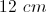and an area of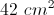. What must be the height of this triangle?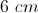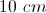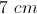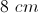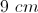Explanation: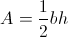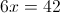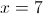All High School Math Resources